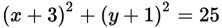# SAT Math Multiple Choice Question 303: Answer and Explanation

### Test Information

Question: 303

3.The graph of the equation above is a circle. What is the area, in square units, of the circle?

• A. 4π
• B. 5π
• C. 16π
• D. 25π